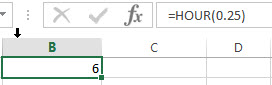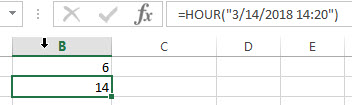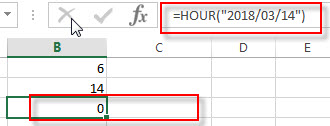# Excel HOUR Function

This post will guide you how to use Excel HOUR function with syntax and examples in Microsoft excel.

### Description

The Excel HOUR function returns the hour of a time value. Or returns an integer value that represent the hour component of a given time. And the return value is between 0-23.

For example, if you pass a time of 13:36 PM into HOUR function, and it will return 13. So you can use this function to extract the hour component from a time value.

The HOUR function is a build-in function in Microsoft Excel and it is categorized as a DATE and TIME Function.

The HOUR function is available in Excel 2016, Excel 2013, Excel 2010, Excel 2007, Excel 2003, Excel XP, Excel 2000, Excel 2011 for Mac.

### Syntax

The syntax of the HOUR function is as below:

`=HOUR (serial_number)`

Where the HOUR function arguments is:

Serial_text -This is a required argument. The time from which you want to extract the hour component. it may be typed as text string within quotation marks (for example, “13:52 PM”), or it can be a decimal numbers(for example, 0.5), or the result of a formula (for example, TIMEVALUE(“13.54PM”))

Note:

• Time values are a portion of a date value and represented by a decimal number (for example, 12:00 PM is represented as 0.5 because it is half of a day).
• A serial date is how the Microsoft Excel stores dates and times and it represents the number of days since 1900-01-01, so the January 1, 1900 date is serial number 1 by default.
• if the serial_number is not a valid Excel time, it will return #VALUE! error.

### Excel HOUR Function Examples

The below examples will show you how to use Excel HOUR Function to return the hour of a given time value.

1# get the hour component of 25% of 24 hours,, enter the following formula in Cell B1.

`=HOUR(0.25)`2# get the hour portion of the date and time value, enter the following formula in Cell B2.

`=HOUR("3/14/2018 14:20")`3# Get the hour portion of the date with no time portion specified, enter the following formula in Cell B3.

`=HOUR("2018/03/14")`so it will be considered 12:00AM or 0 hours.

### Related Functions

• Excel MINUTE Function
The Excel MINUTE function returns the minutes of a time value. And the minutes is an integer number from 0 to 59. The syntax of the MINUTE function is as below:=MINUTE (serial_number)…
• Excel SECOND Function
The Excel SECOND function returns the seconds of a time value. Or returns an integer value that represent the second component of a given excel time. And the return value is between 0-59.The syntax of the SECOND function is as below:=SECOND (serial_number)…
• Excel DAY function
The Excel DAY function returns a day of a date (from 1 to 31). The DAY function is a build-in function in Microsoft Excel and it is categorized as a DATE and TIME Function.The syntax of the DAY function is as below:= DAY (date_value)
• Excel YEAR function
The Excel YEAR function returns a four-digit year from a given date value, the year is returned as an integer ranging from 1900 to 9999. The syntax of the YEAR function is as below:=YEAR (serial_number)…
• Excel MONTH function
The Excel MONTH function returns the month of a date represented by a serial number. And the month is an integer number from 1 to 12. The syntax of the MONTH function is as below:=MONTH (serial_number)…
• Excel TIMEVALUE Function
The Excel TIMEVALUE function returns the decimal number of the time represented by a text string. so it will convert a time represented by a text string into an Excel time value.The syntax of the TIMEVALUE function is as below:=TIMEVALUE (time_text)…